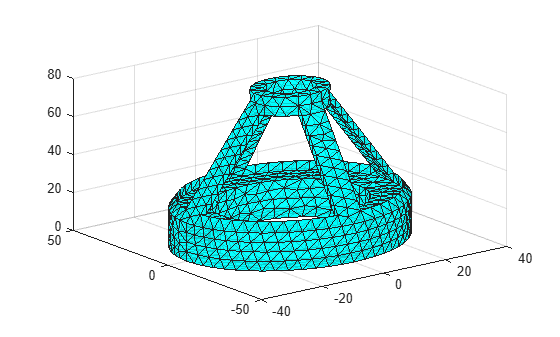# freeBoundary

Free boundary facets

## Syntax

``F = freeBoundary(TR)``
``[F,P] = freeBoundary(TR)``

## Description

example

````F = freeBoundary(TR)` returns the free boundary facets of the triangles or tetrahedra in `TR`. A facet in `TR` is on the free boundary if it is referenced by only one triangle or tetrahedron.```

example

````[F,P] = freeBoundary(TR)` also returns a matrix containing the vertices of the free boundary facets.```

## Examples

collapse all

You can use the `freeBoundary` function to highlight the outer edges of a 2-D Delaunay triangulation.

Create a triangulation from a 2-D set of points.

```rng('default') x = rand([20,1]); y = rand([20,1]); TR = delaunayTriangulation(x,y);```

Find the free boundary edges.

`F = freeBoundary(TR);`

Plot the triangulation and highlight the free boundary edges in red.

```triplot(TR) hold on plot(x(F),y(F),'-r','LineWidth',2)```You can use the `freeBoundary` function to extract the facets of a 3-D triangulation that cover the surface of an object.

```load tetmesh TR = triangulation(tet,X);```

Compute the boundary triangulation.

`[F,P] = freeBoundary(TR);`

Plot the boundary triangulation.

```trisurf(F,P(:,1),P(:,2),P(:,3), ... 'FaceColor','cyan','FaceAlpha',0.8);```## Input Arguments

collapse all

Triangulation representation, specified as a scalar `triangulation` or `delaunayTriangulation` object.

Data Types: `triangulation` | `delaunayTriangulation`

## Output Arguments

collapse all

Triangulation connectivity list, returned as a matrix.

If you call `freeBoundary` with one output argument, then the elements of `F` are row numbers in the `Points` property of the input triangulation. If you call `freeBoundary` with two output arguments, then the elements of `F` are row numbers in `P`.

Data Types: `double`

Free boundary points, returned as a matrix containing the coordinates of the vertices of the free boundary facets. Each row of `P` contains the coordinates of a vertex.

Data Types: `double`